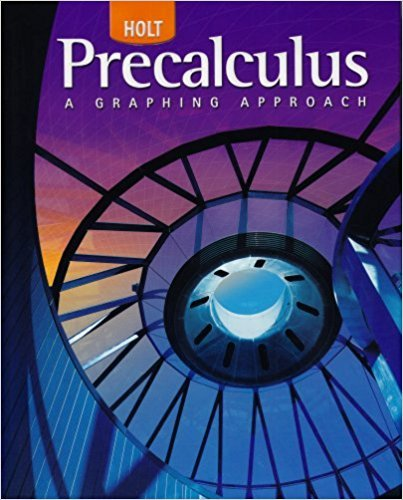×

×

# Solutions for Chapter 12.2: Systems and Matrices## Full solutions for Precalculus | 1st Edition

ISBN: 9780030416477Solutions for Chapter 12.2: Systems and Matrices

Solutions for Chapter 12.2
4 5 0 237 Reviews
29
5
##### ISBN: 9780030416477

Since 10 problems in chapter 12.2: Systems and Matrices have been answered, more than 26196 students have viewed full step-by-step solutions from this chapter. This textbook survival guide was created for the textbook: Precalculus, edition: 1. This expansive textbook survival guide covers the following chapters and their solutions. Precalculus was written by and is associated to the ISBN: 9780030416477. Chapter 12.2: Systems and Matrices includes 10 full step-by-step solutions.

Key Calculus Terms and definitions covered in this textbook
• Deductive reasoning

The process of utilizing general information to prove a specific hypothesis

• Differentiable at x = a

ƒ'(a) exists

• Divergence

A sequence or series diverges if it does not converge

• Division

a b = aa 1 b b, b Z 0

• Infinite limit

A special case of a limit that does not exist.

• Inverse composition rule

The composition of a one-toone function with its inverse results in the identity function.

• Inverse secant function

The function y = sec-1 x

• Inverse sine function

The function y = sin-1 x

• Invertible linear system

A system of n linear equations in n variables whose coefficient matrix has a nonzero determinant.

See Polynomial function in x.

• Length of a vector

See Magnitude of a vector.

• Linear equation in x

An equation that can be written in the form ax + b = 0, where a and b are real numbers and a Z 0

• Logarithmic re-expression of data

Transformation of a data set involving the natural logarithm: exponential regression, natural logarithmic regression, power regression

• Parametric curve

The graph of parametric equations.

• Piecewise-defined function

A function whose domain is divided into several parts with a different function rule applied to each part, p. 104.

A procedure for fitting a quadratic function to a set of data.

• Re-expression of data

A transformation of a data set.

• Reflection

Two points that are symmetric with respect to a lineor a point.

• Response variable

A variable that is affected by an explanatory variable.

• Standard form: equation of a circle

(x - h)2 + (y - k2) = r 2